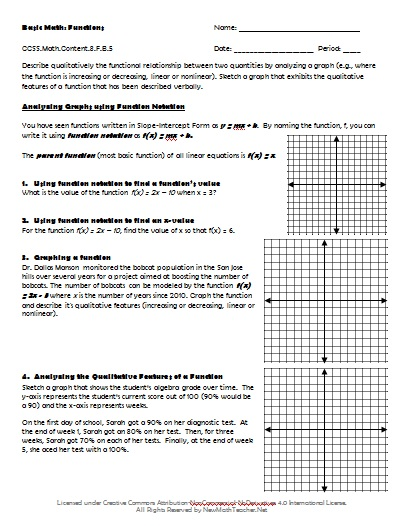# Engaging Math Lessons.

Please browse our selection of mathematics lessons sorted by subject and current common core standards for improving math achievement for every student.

Below are a set of lesson plans and worksheets for Grade 8 Math that correspond to Common Core concepts and help students solve real-world problems. Lesson previews provide a quick glimpse at the provided materials.

• ### Concept:

Functions
• Common Core Standard: CCSS.Math.Content.8.F.A.1
• Description: Understand that a function is a rule that assigns to each input exactly one output. The graph of a function is the set of ordered pairs consisting of an input and the corresponding output.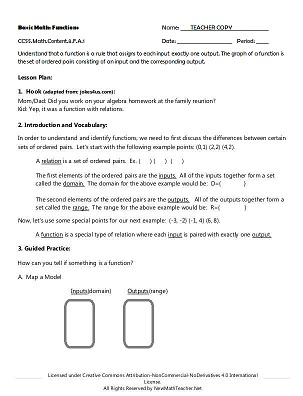• ### Concept:

Functions
• Common Core Standard:CCSS.Math.Content.8.F.A.2
• Description: Compare properties of two functions each represented in a different way (algebraically, graphically, numerically in tables, or by verbal descriptions).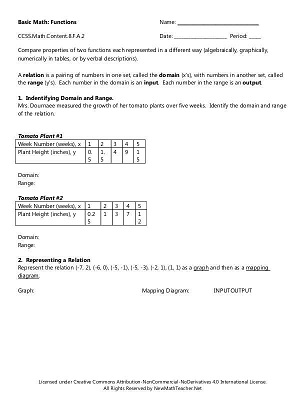• ### Concept:

Functions
• Common Core Standard:CCSS.Math.Content.8.F.A.3
• Description: Interpret the equation y = mx + b as defining a linear function, whose graph is a straight line; give examples of functions that are not linear.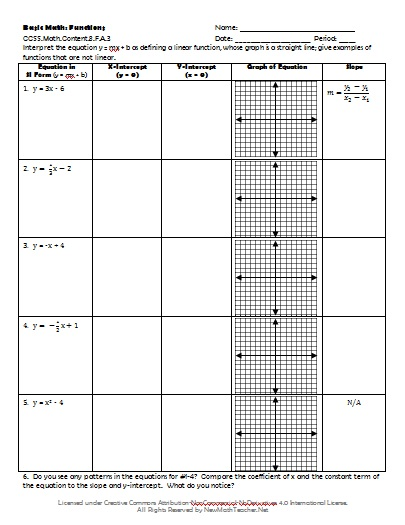• ### Concept:

Functions
• Common Core Standard:CCSS.Math.Content.8.F.B.4
• Description: Construct a function to model a linear relationship between two quantities. Determine the rate of change and initial value of the function from a description of a relationship or from two (x, y) values, including reading these from a table or from a graph. Interpret the rate of change and initial value of a linear function in terms of the situation it models, and in terms of its graph or a table of values.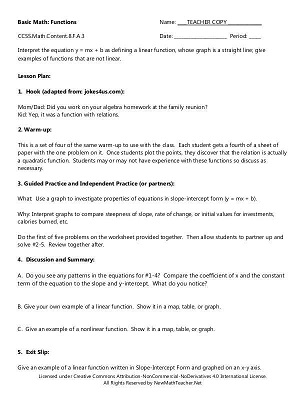• ### Concept:

Functions
• Common Core Standard:CCSS.Math.Content.8.F.B.5
• Description: Describe qualitatively the functional relationship between two quantities by analyzing a graph (e.g., where the function is increasing or decreasing, linear or nonlinear). Sketch a graph that exhibits the qualitative features of a function that has been described verbally.